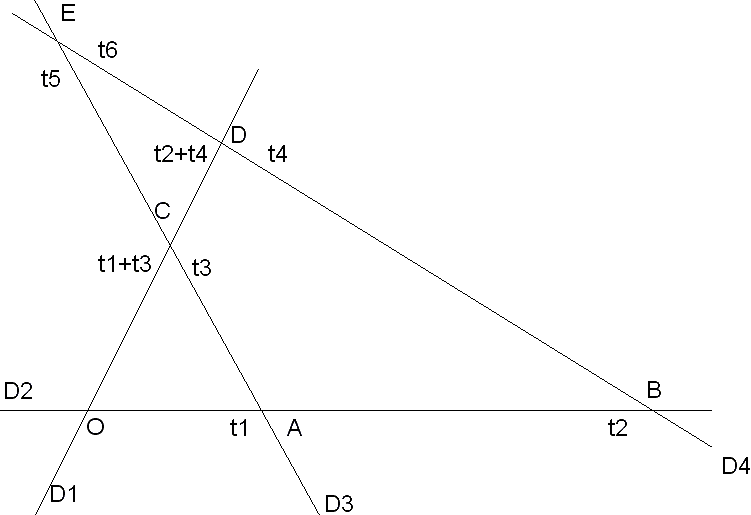## Solution to the $4\,$ Travelers problem

The following is based on the solution to the Four Travelers problem sent to me by Michel Cabart. To remind, here is the problem:

Four roads on a plane, each a straight line, are in general position. Along each road walks a traveler at a constant speed. Their speeds, however, may not be the same. It's known that traveler #1 met with Travelers #2, #3, and #4. #2, in turn, met #3 and #4 and, of course, #1. Show that #3 and #4 have also met.

Michel's solution refers to the following diagram:The four roads are denoted $D_{1},\,$ $D_{2},\,$ $D_{3},\,$ and $D_{4}.\,$ The intersections $O,\,$ $A,\,$ $B,\,$ $C,\,$ $D,\,$ $E\,$ are defined by

$O = D_{1} \times D_{2}, A = D_{2} \times D_{3}, B = D_{2} \times D_{4},\\ C = D_{1} \times D_{3}, D = D_{1} \times D_{4}, E = D_{3} \times D_{4}.$

Accordingly, we assume that the first traveler moves in the direction from $O\,$ to $C\,$ to $D,\,$ the second from $O\,$ to $A\,$ to $B,\,$ the third from $A\,$ to $C\,$ to $E,\,$ and the forth from $B\,$ to $D\,$ to $E.\,$ For every traveler, i.e., for every traveler, we set up a private time frame. We assume that $O\,$ serves as $t = 0\,$ on roads $D_{1}\,$ and $D_{2},\,$ on road $D_{3}\,$ $t = 0\,$ at $A,\,$ and, for $D_{4},\,$ $t = 0\,$ at $B.\,$

Denoting their speeds $v_{1}, \ldots, v_{4},\,$ we time the progress of each of the travelers in their private time frames so that

$OA = t_{1}v_{2}, OB = t_{2}v_{2},\\ AC = t_{3}v_{3}, OE = t_{5}v_{3},\\ BD = t_{4}v_{4}, BE = t_{6}v_{4}.$

Now, after travelers 1 and 2 part after a meeting at $O,\,$ 1 continues towards $C\,$ where he is going to meet traveler 3. Traveler 2 proceeds towards $A\,$ where he greets traveler 3 after $t_{1}\,$ (time units). Traveler 3 starts his time count from this point. It takes him $t_{3}\,$ to get to $C\,$ where he meets traveler 1. Thus the latter spent $t_{1} + t_{3}\,$ on the road between intersections $O\,$ and $A.\,$ In a similar vein, the first traveler spends $t_{2} + t_{4}\,$ between $O\,$ and $D.$

More generally, for every triangle formed by the roads, the condition that all its vertices serve as a meeting place for respective travelers is equivalent to an identity between time intervals. In particular, in order to prove that travelers 3 and 4 meet in $E,\,$ we only need to show that

$t_{5} + (t_{2} - t_{1}) = t_{6}, \,\text{or}\, t_{5} - t_{6} = t_{2} - t_{1}.$

Applying Menelaus' Theorem to triangle $ABE\,$ and line $OCD\,$ yields:

$OA\cdot CE\cdot DB = OB\cdot CA\cdot DE,$

or

$[t_{1}v_{2}]\cdot [(t_{5} - t_{3})v_{3}]\cdot [t_{4}v_{4}] = [t_{2}v_{2}]\cdot [t_{3}v_{3}]\cdot [(t_{6} - t_{4})v_{4}],$

which simplifies to

(1)

$t_{1}\cdot (t_{5} - t_{3})\cdot t_{4} = t_{2}\cdot t_{3}\cdot (t_{6} - t_{4}).$

Similarly, applying Menelaus' Theorem to $ΔCED\,$ and line $OAB\,$ we get

$OD\cdot AC\cdot BE = OC\cdot AE\cdot BD$

or

(2)

$(t_{2} + t_{4})\cdot t_{3}\cdot t_{6} = (t_{1} + t_{3})\cdot t_{5}\cdot t_{4}$

(1) and (2) can be manipulated into

$t_{1}\cdot t_{4}\cdot t_{5} ñ t_{2}\cdot t_{3}\cdot t_{6} = t_{3}\cdot t_{4}\cdot (t_{1} ñ t_{2})$

and

$t_{1}\cdot t_{4}\cdot t_{5} ñ t_{2}\cdot t_{3}\cdot t_{6} = t_{3}\cdot t_{4}\cdot (t_{6} ñ t_{5}),$

which obviously yields the desired result.